Author: Oscar Cronquist Article last updated on April 02, 2018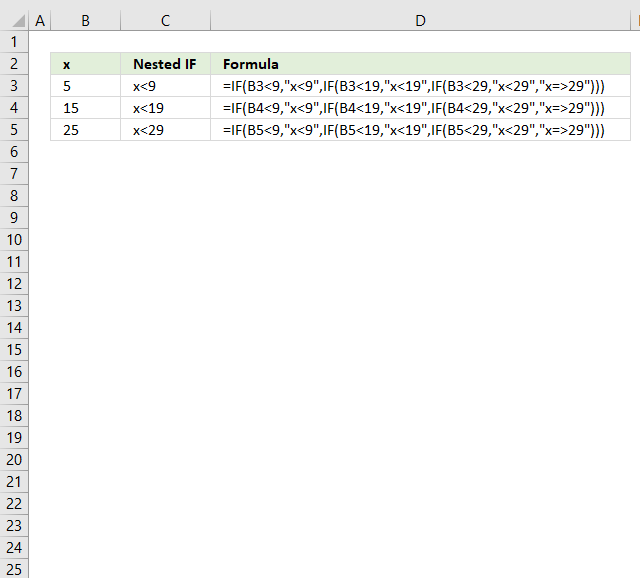Nested IF statements in a formula are multiple combined IF functions so more conditions and outcomes become possible. They all are more or less complicated to read and thankfully there are better alternatives.

You are allowed to use up to 254 nested IF statements but before you do that make sure you read this article, I promise you it will save you a lot of time.

This article will provide step by step tutorials for your specific scenario. If you can't find what you are looking for here please comment and I will add the missing part.

### Nested IF functions between two numbers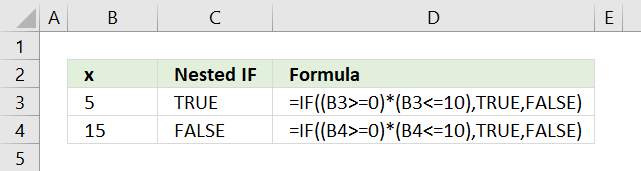You don't need to use multiple IF statements if you want to check that cell value is between two values, it is enough to simply use two logical expressions in the first argument.

The following formula returns TRUE if the value in cell B3 is equal to or greater than 0 and is equal to or smaller than 10.

=IF((B3>=0)*(B3<=10), TRUE, FALSE)

Each logical expression is encapsulated with parentheses, this makes sure that the comparisons are made before multiplying the expressions. In other words, the parentheses determine the order of calculation.

The asterisk between the logical expressions means that both logical expressions must return TRUE for the logical test argument to return TRUE.

### Nested IF statements with ranges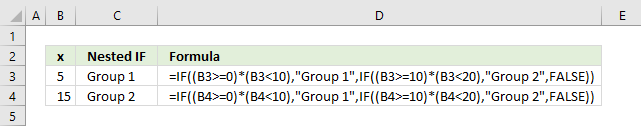The picture above shows you nested IF statements that allow you to return different outcomes depending on the value in column B.

A value equal to or greater than 0 and smaller than 10 is in "Group 1".

A value equal to or greater than 10 and smaller than 20 returns "Group 2".

Imagine that you have 5 different groups, you now need to add 3 more nested IF statements. The formula grows considerably, however, there is a smart alternative to nested IF statements.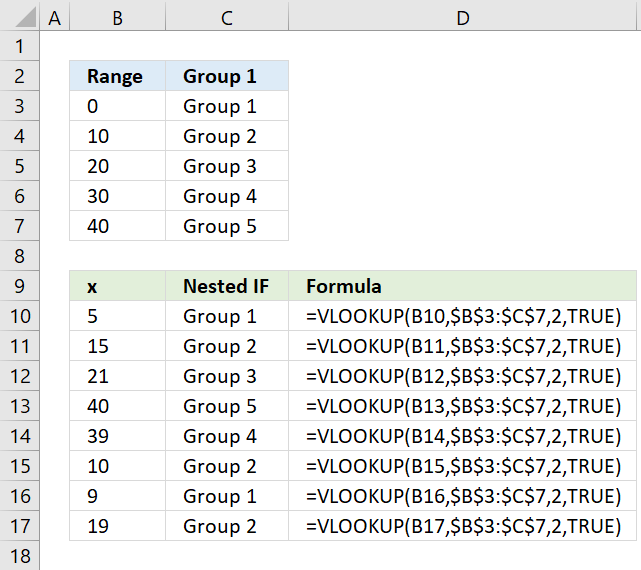The VLOOKUP lets you easily group numbers using a simple function instead of constructing a megaformula that is hard to follow and troubleshoot.

Need more groups? No, problem. Add groups to the first table and then adjust the cell reference in the second argument in the VLOOKUP function.

=VLOOKUP(B10, \$B\$3:\$C\$7, 2, TRUE)

Note, the first table must have the first column sorted from small to large. Make sure you use TRUE in the fourth VLOOKUP argument. This means that it only needs an approximate match.

### Nested IF functions with text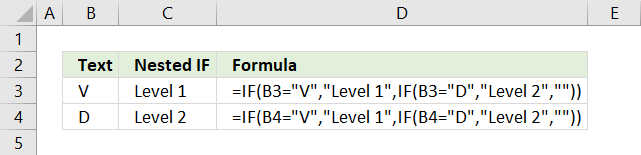These nested IF statements check if a value is equal to "V" and returns "Level 1", if false it continues to the next IF statement and there check if the value is equal to "D". If it is it returns "Level 2"

You can use the VLOOKUP function in this case as well as an alternative to nested IF functions.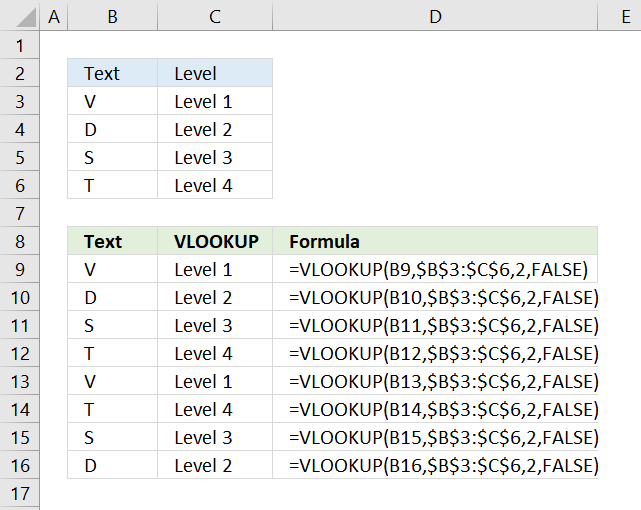You need to find an exact match in this case so change the fourth VLOOKUP argument to FALSE.

=VLOOKUP(B9,\$B\$3:\$C\$6,2,FALSE)

The VLOOKUP function looks for the value in the first column (B3:C6) and returns the corresponding value in the second column. That is why I use 2 in the third VLOOKUP argument.

Use VLOOKUP to calculate discounts, commissions, tariffs, charges, shipping costs, packaging expenses or bonuses

Have you ever tried to build a formula to calculate discounts depending on price? The VLOOKUP function is much easier to […]

### Nested if statements with dates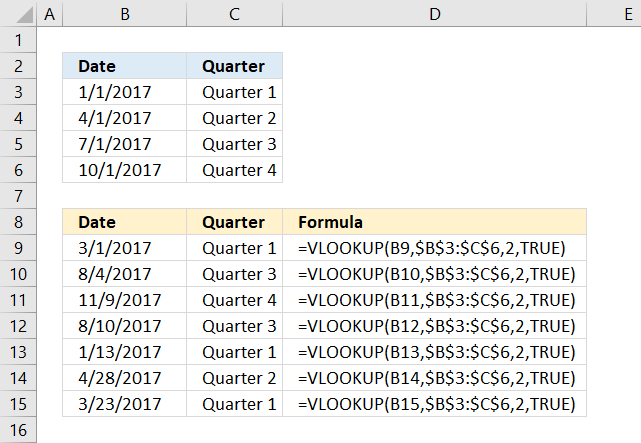The above image demonstrates how to use multiple date ranges with a short and simple VLOOKUP function. A date range consists of two dates, since these date ranges are contiguous the end date also represents the start date for the next range.

The following picture shows how to setup non-contiguous date ranges.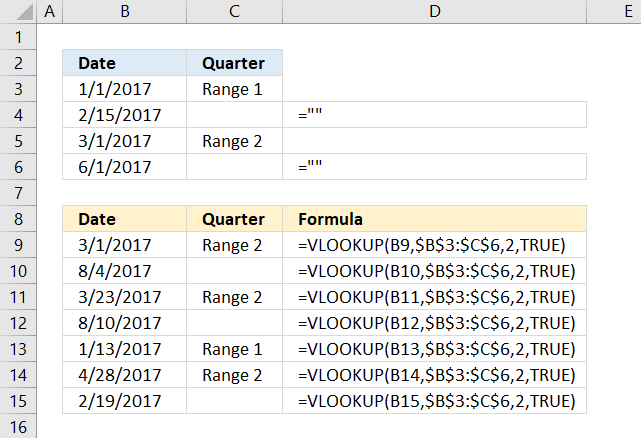The first cell range is between 1/1/2017 and 2/15/2017. The second cell range is between 3/1/2017 and 6/1/2017.

The VLOOKUP function returns 0 if a cell is empty, to prevent that type ="" and it will return a blank cell.